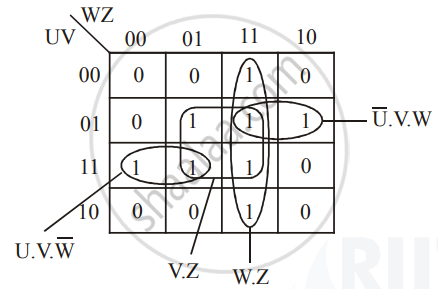Share

# Reduce the Following Boolean Expression to Its Simplest Form Using K-map: G(U, V,W, Z) = Sum(3,5 , 6 , 7 , 11 , 12 ,13, 15) - CBSE (Arts) Class 12 - Computer Science (Python)

ConceptReducing Boolean Expression (SOP and POS) to Its Minimal Form

#### Question

Reduce the following Boolean Expression to its simplest form using K-Map:

G(U, V,W, Z) = sum(3,5 , 6 , 7 , 11 , 12 ,13, 15)

#### Solution

G(U, V,W, Z) = sum(3,5 , 6 , 7 , 11 , 12 ,13, 15)

= W.Z + V.Z + barU.V.W + U.V.bar(W)Is there an error in this question or solution?

#### APPEARS IN

Solution Reduce the Following Boolean Expression to Its Simplest Form Using K-map: G(U, V,W, Z) = Sum(3,5 , 6 , 7 , 11 , 12 ,13, 15) Concept: Reducing Boolean Expression (SOP and POS) to Its Minimal Form.
S